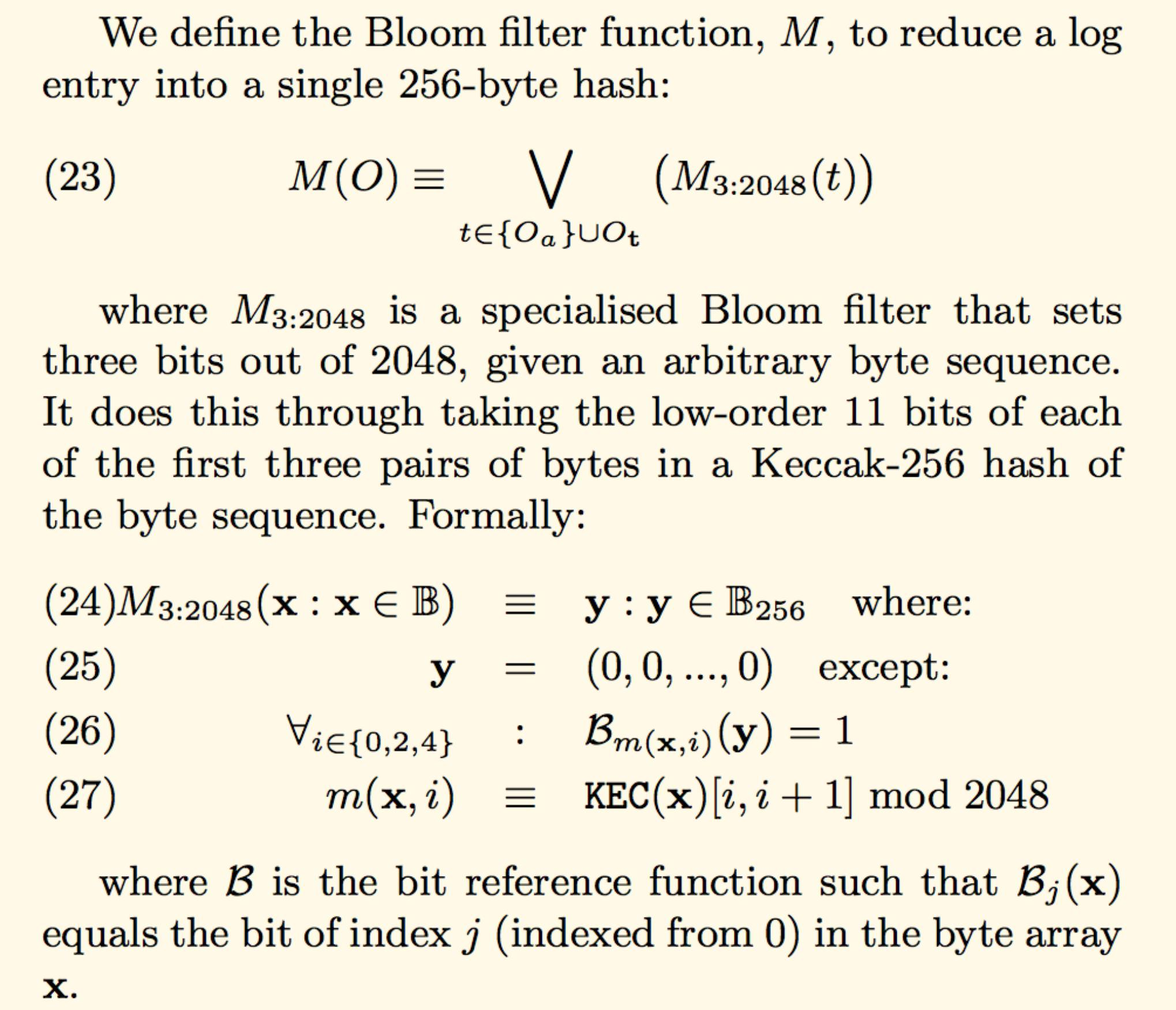# Bloom filters in Ethereum### Background

Bloom filters are compact data structures for probabilistic representation of a set in order to support membership queries (i.e. queries that ask: “Is element X in set Y?”).

An empty Bloom filter is a bit array of m bits, all set to 0. There must also be k different hash functions defined, each of which maps or hashes some set element to one of the m array positions, generating a uniform random distribution. Typically, k is a constant, much smaller than m, which is proportional to the number of elements to be added; the precise choice of k and the constant of proportionality of m are determined by the intended false positive rate of the filter.

To add an element, feed it to each of the k hash functions to get k array positions. Set the bits at all these positions to 1.

To query for an element (test whether it is in the set), feed it to each of the k hash functions to get k array positions. If any of the bits at these positions is 0, the element is definitely not in the set – if it were, then all the bits would have been set to 1 when it was inserted. If all are 1, then either the element is in the set, or the bits have by chance been set to 1 during the insertion of other elements, resulting in a false positive. In a simple Bloom filter, there is no way to distinguish between the two cases, but more advanced techniques can address this problem

Pros:

• space efficient

Cons:

• can’t store an associated object
• deletions are not allowed
• small false positive probability

Applications:

• cache sharing
• query filtering and routing
• blockchain (logs)

### Ethereum bloom filter function

Here’s excerpt from the yellow paper:If you didn’t understand anything, no worries, I will try to describe its implementation in the next section.

### Elixir implementation

``````  @spec bloom(integer(), binary()) :: integer()
def bloom(data, number \\ 0) do
bits =
data
|> sha3_hash            \\ (1)
|> bit_numbers          \\ (2)

end

``````

The bloom/2 method accepts 2 aguments:

• data - binary. a new object to add to the bloom filter
• number - integer. the bloom filter. default value is zero (for new filter)

Bloom filter generation can be divided into three parts:

• calculate sha3 hash of an input data (1)
• get three bit indices from this hash (2)
• set these indices to one in the filter (3)
``````  @spec bit_numbers(binary()) :: [integer()]
defp bit_numbers(hash) do
{result, _} =
1..3
|> Enum.reduce({[], hash}, fn(_, acc) ->

{[new_bit|bits], tail}
end)

result
end
``````

The bit_numbers/1 method gets indices from the low order 11-bits of the first three double-bytes of the SHA3 hash.

``````  @spec add_bits(integer(), [integer()]) :: integer()
bits
|> Enum.reduce(bloom_number, fn(bit_number, bloom) ->
bloom ||| (1 <<< bit_number)
end)
end
``````

The last thing left to do is to define method to check if filter has an object:

``````  @spec contains?(integer(), binary()) :: boolean()
def contains?(current_bloom, val)
when is_integer(current_bloom) and
is_binary(val) do
temp_bloom = bloom(val)

(temp_bloom &&& current_bloom) == temp_bloom
end
``````

Now we can use our Bloom filter:

``````iex(1)> filter = EthBloom.create("rock")
364236115780354413527177740824718248475191169123433179704674083584030171707548895141763338864017157468044931245187476551639917006481686358559826056819612140499456696964058447681377941080842953023782344796365486712958202642967905678562795813182653349819408730761190197772532753708011416878102770310284058867224260870159690230717337345574083724951534664321152788810106636281468311475567122070065682554961594780791945980493299780947753165983844578885074727735505366654109046841451940478976

364236115780354413527177740824718248475191169123433179704674083584030171707548895141763338864017157468044931245187476551639917008583551526575664755314165081912131926893248480084782472542419712838325886207711387518315201817289948316059274529230320334129359156724892064138319603709966466517388006639868069257972765204989827007471817298541374196685568760261999794300328280707725224364790299899128819784076530509337252429855458993811873518002173094917629136344228828519818452981281942732800

iex(3)> EthBloom.contains?(new_filter, "punk")
false

iex(4)> EthBloom.contains?(new_filter, "rock")
true

iex(5)> EthBloom.contains?(new_filter, "blues")
true
``````

Described code has a GitHub repository - https://github.com/ayrat555/eth_bloom